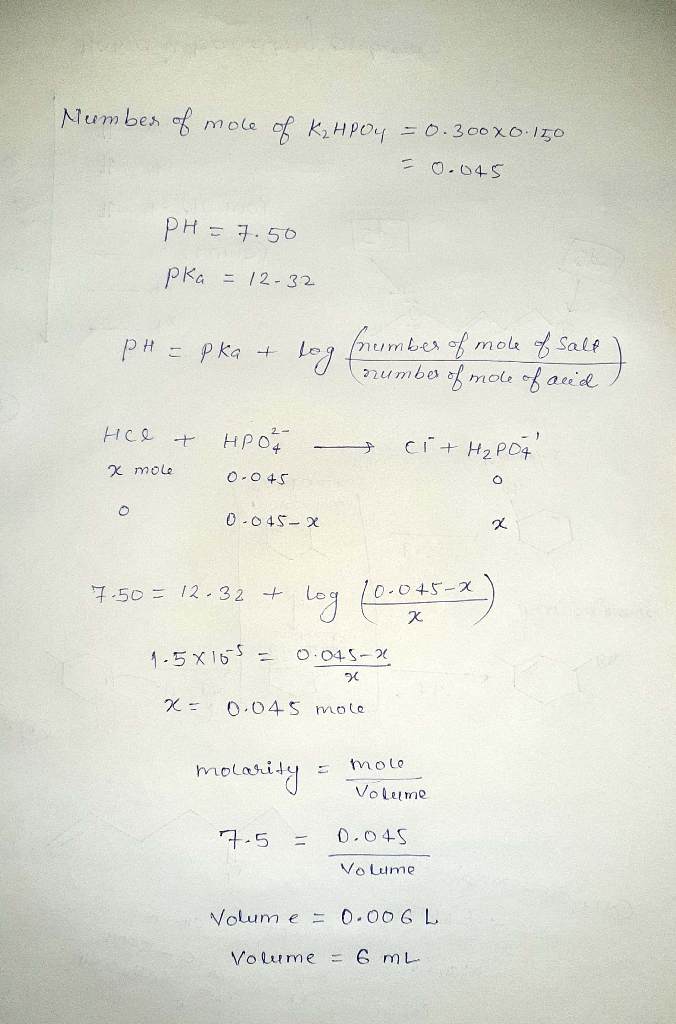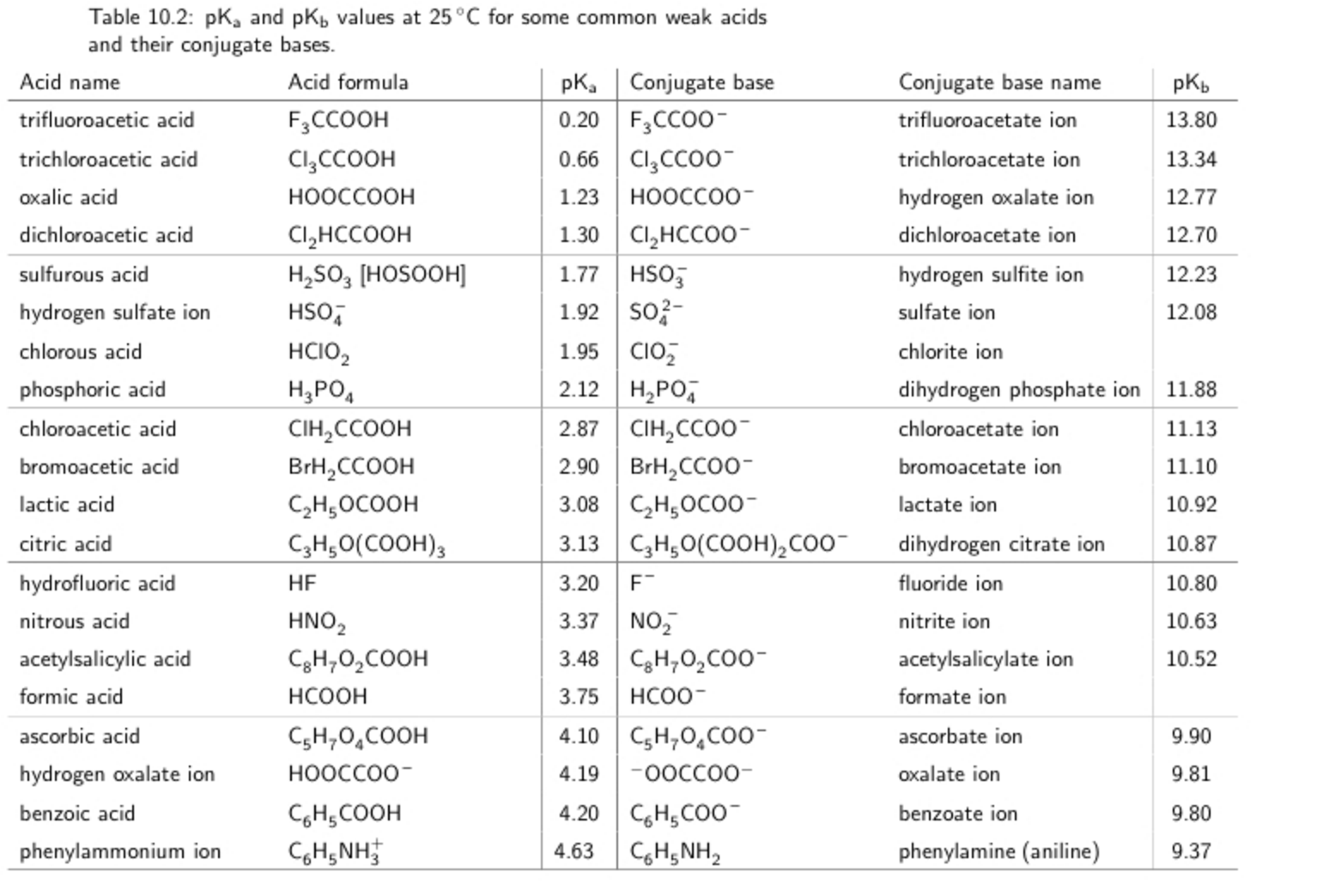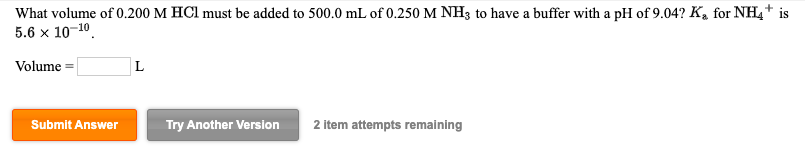Question

# What volume (to the nearest 0.1 mL) of 7.50-M HCl must be added to 0.300 L...

What volume (to the nearest 0.1 mL) of 7.50-M HCl must be added to 0.300 L of 0.150-M K2HPO4 to prepare a pH = 7.50 buffer?

(pKa of hydrogen phosphate ion = 12.32)#### Earn Coins

Coins can be redeemed for fabulous gifts.

Similar Homework Help Questions
• ### Determine the volume (in mL) of 0.223 M hydrochloric acid (HCl) that must be added to...Determine the volume (in mL) of 0.223 M hydrochloric acid (HCl) that must be added to 65.4 mL of 0.0842 M sodium hydrogen phosphate (Na2HPO4) to yield a pH of 7.13. attached is the pka table that we were given

• ### What volume of 0.200 M HCl must be added to 500.0 mL of 0.250 M NH3...

What volume of 0.200 M HCl must be added to 500.0 mL of 0.250 M NH3 to have a buffer with a pH of 8.81? Ka for NH4+ is 5.6x10-10. Volume =. L

• ### What volume (in mL) of 0.150 M NaOH must be added to 100.0 mL of a...

What volume (in mL) of 0.150 M NaOH must be added to 100.0 mL of a 0.0500 F solution of fumaric acid (trans-butenedioic acid) to make a buffer of pH 4.50? Ka1 of Fumeric acid = 8.85x10^-4   pKa = 3.05 Ka2 of Fumeric acid = 3.21x10-5

• ### How many grams (to the nearest 0.01 g) of NH4Cl (Mm = 53.49 g/mol) must be...

How many grams (to the nearest 0.01 g) of NH4Cl (Mm = 53.49 g/mol) must be added to 550. mL of 0.976-M solution of NH3 in order to prepare a pH = 9.15 buffer? What volume (to the nearest 0.1 mL) of 4.00-M NaOH must be added to 0.600 L of 0.300-M HNO2 to prepare a pH = 3.50 buffer?

• ### What volume of 0.200 M HCl must be added to 500.0 mL of 0.250 M NH3...What volume of 0.200 M HCl must be added to 500.0 mL of 0.250 M NH3 to have a buffer with a pH of 9.04? K, for NH4* is 5.6 x 10-10 Volume = L Submit Answer Try Another Version 2 item attempts remaining

• ### A. How many grams of ammonium chloride must be added to 0.80 L of 1.7 M...

A. How many grams of ammonium chloride must be added to 0.80 L of 1.7 M ammonia to prepare a pH = 10.29 buffer? B. How many milliliters of 2.9 M HCl must be added to 2.6 L of 0.17 M K2HPO4 to prepare a pH = 7.44 buffer? C. What is the pH of a solution prepared by mixing 24 mL of 0.050 M Ba(OH)2 and 39 mL of 0.44 M KOH? (This is just a mixing problem: simply...

• ### What concentration of HCl will have a pH of 5.0? b) What concentration of acetic acid will have a pH of 5.0? (For acetic...

What concentration of HCl will have a pH of 5.0? b) What concentration of acetic acid will have a pH of 5.0? (For acetic acid, pKa=4.76) c) What concentration of phosphoric acid will have a pH of 5.0? (For H3PO4, pKa1 = 2.12, pKa2 = 7.21, pKa3 =12.32) d) What is the pH of a 10 mM solution of phosphoric acid? e) How much 1.0 M NaOH must be added to 100 ml of 10 mM H3PO4 to raise the...

• ### 7. In each of the following questions, assume that there is no volume change when HCl is added to water in part (a) or t...

7. In each of the following questions, assume that there is no volume change when HCl is added to water in part (a) or the phosphate buffer in part (c). (a) Calculate the pH when 0.021 moles of HCl are added to 1.000 liter of water. (b) What is the difference between the pH of pure water (pH 7.00) and the pH of the solution after HCl was added? (c) Calculate the pH when 0.021 moles of HCl are added...

• ### What volume of 0.089 M HCl must be added to 765 mL of 0.123 M Na2CO3...

What volume of 0.089 M HCl must be added to 765 mL of 0.123 M Na2CO3 to obtain a 9.7 pH?

• ### (a) What is the pH of a solution that contains 0.100 M HClO and 0.300 M...

(a) What is the pH of a solution that contains 0.100 M HClO and 0.300 M KClO? (b) If 50.00 mL of 0.100 M HCl is added to 1.00 L of the above buffer, what is the new pH? (b) If 50.00 mL of 0.100 M HCl is added to 1.00 L of the above buffer, what is the new pH?